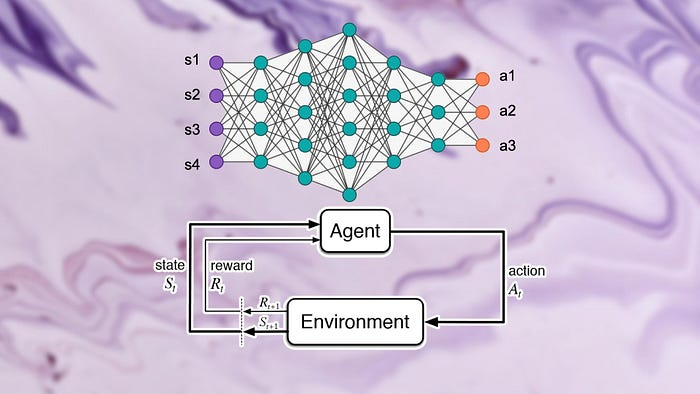# Demystifying Machine Learning Algorithm Evaluation: A Comprehensive Guide to Assessing Model Performance# Introduction

Machine learning (ML) has revolutionized various fields, from healthcare to finance and beyond. However, the success of ML applications hinges on the appropriate selection and evaluation of algorithms. A model’s performance can be greatly affected by the choice of the algorithm, and understanding how to evaluate these algorithms is a crucial aspect of machine learning. This comprehensive guide will delve into the ins and outs of evaluating machine learning algorithms.

# Understanding Algorithm Evaluation

Evaluating a machine learning algorithm involves assessing how well the algorithm can predict new, unseen data based on its learning from the training data. The objective is to find an algorithm that generalizes well, rather than one that performs optimally on the training data but poorly on new data, a problem known as overfitting.

An effective evaluation helps in comparing different algorithms, selecting the most appropriate one for the task at hand, and tuning the algorithm’s hyperparameters for optimal performance.

# Establishing a Baseline

A baseline provides a point of reference to compare the performance of different machine learning algorithms. Typically, a simple and well-understood algorithm is used to establish a baseline. The objective of the machine learning project is then to develop a model that outperforms this baseline. For instance, in a binary classification problem, a common baseline algorithm is the “Zero Rule” algorithm, which predicts the most common class in the training dataset.

# Evaluating With Resampling Methods

Resampling methods are statistical techniques that involve repeatedly drawing samples from a training dataset and refitting a model of interest on each sample in order to obtain additional information about the fitted model. These techniques allow us to estimate how well an algorithm is likely to perform on unseen data. Some common resampling methods include:

Train/Test Split: This involves splitting the dataset into a training set and a test set. The model is trained on the training set and evaluated on the test set. While simple and fast, this method can have high variance, meaning that different splits might result in significantly different results.

k-fold Cross-Validation: In this method, the dataset is divided into k subsets. The model is trained on k-1 subsets and tested on the remaining one. This process is repeated k times, with each subset used exactly once as the test set. The performance of the model is then averaged over the k runs. This method provides a more reliable estimate of performance than the train/test split, but it’s also more computationally intensive.

Stratified k-fold Cross-Validation: This is a variation of k-fold cross-validation that is used when the data is imbalanced (i.e., one class has many more examples than another). It ensures that each fold contains roughly the same proportions of the different classes as the whole dataset.

Leave-One-Out Cross-Validation: This is a special case of k-fold cross-validation where k equals the total number of observations in the dataset. It provides a very robust estimate of performance, but it’s also the most computationally intensive.

# Metrics for Algorithm Evaluation

The choice of evaluation metric should align with the business objective of the machine learning project. Different problems will require different metrics. Some common ones include:

Accuracy: This is the proportion of correct predictions out of the total predictions and is a common metric for classification problems.

Precision, Recall, and F1-Score: These are useful for binary classification problems, especially when the data is imbalanced.

Mean Absolute Error and Mean Squared Error: These are common metrics for regression problems.

Area Under ROC Curve (AUC-ROC): This is used for binary classification problems and provides a good

measure of a model’s performance across all possible classification thresholds.

# Comparative Studies

To determine the best algorithm for your problem, you might need to perform a comparative study. This involves evaluating multiple algorithms on the same dataset using the same resampling method and metric. The algorithm that performs the best on average is then selected as the most suitable for the problem.

# Conclusion

Evaluating machine learning algorithms is a critical step in any machine learning project. It provides a way to assess the performance of an algorithm, compare different algorithms, and select the best one for the problem at hand.

The process of evaluation involves establishing a baseline, using resampling methods to estimate the performance of the algorithm, selecting an appropriate metric, and possibly performing a comparative study. While this process can be complex, it’s also crucial for the success of your machine learning project.

By understanding how to effectively evaluate machine learning algorithms, you can make informed decisions that lead to more accurate and reliable models, ultimately driving the success of your machine learning applications.

# Personal Career & Learning Guide for Data Analyst, Data Engineer and Data Scientist

## Applied Machine Learning & Data Science Projects and Coding Recipes for Beginners

A list of FREE programming examples together with eTutorials & eBooks @ SETScholars

# Projects and Coding Recipes, eTutorials and eBooks: The best All-in-One resources for Data Analyst, Data Scientist, Machine Learning Engineer and Software Developer

Topics included:Classification, Clustering, Regression, Forecasting, Algorithms, Data Structures, Data Analytics & Data Science, Deep Learning, Machine Learning, Programming Languages and Software Tools & Packages.
(Discount is valid for limited time only)## Find more … …

Learn Keras by Example – k-Fold Cross-Validating Neural Networks

How to Evaluate the Performance Of Deep Learning Models in Python

Machine Learning for Beginners – Evaluate the Performance of Deep Learning Models in Keras using Python